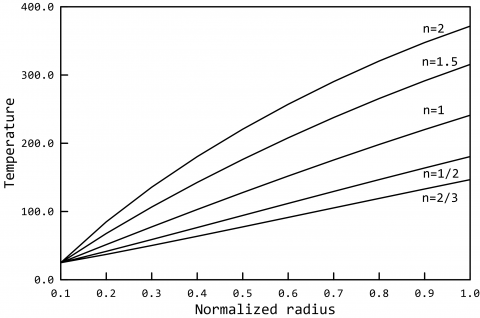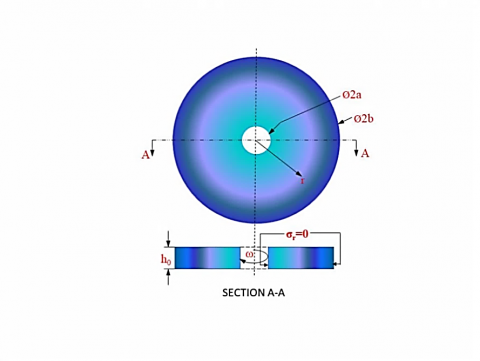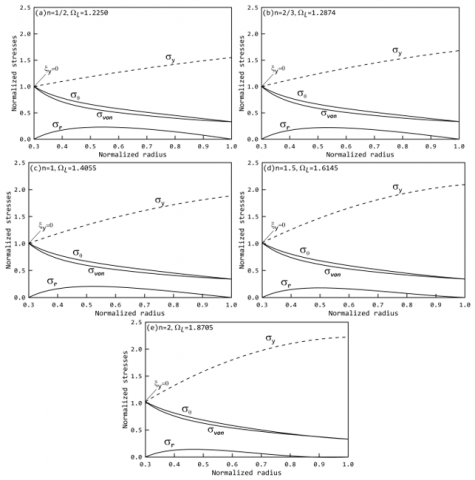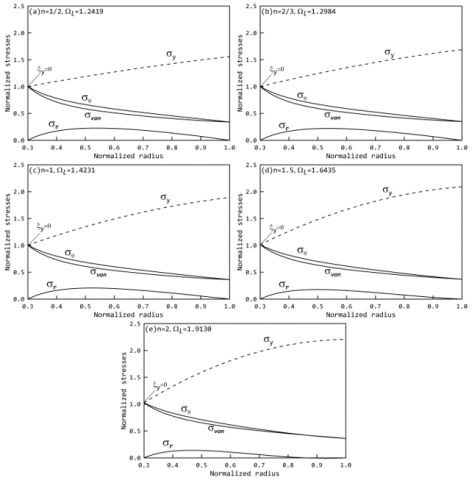# Limit Elastic Analysis of E-FGM Rotating Disk with Temperature Dependent Mechanical Properties

Limit Elastic Analysis of E-FGM Rotating Disk with Temperature Dependent Mechanical Properties
Department of Mechanical Engineering, National Institute of Technology Raipur (C.G) 492010, India

Department of Mechanical Engineering, Jadavpur University, Kolkata 700032, India

Corresponding Author Email:
Page:
634-640
|
DOI:
https://doi.org/10.18280/mmep.060419
19 March 2019
|
Accepted:
11 December 2019
|
Published:
24 December 2019
| Citation

OPEN ACCESS

Abstract:

In present work, exponential law based grading of composition is considered for high speed rotating disks and Young’s modulus is calculated for two cases namely; temperature dependent and temperature independent applications. In temperature dependent applications, Young’s modulus is assumed to vary with temperature as well throughout the spatial coordinates of the disk. The effective Young’s modulus of FGM is then calculated using modified rule of mixture. Limit elastic analysis is performed by using variational principle. Results show the effect of temperature over stresses and obtained limit elastic speed. It is observed that limit speed first increases with an increase in aspect ratio and starts decreasing after reaching a critical value. Limit speed also increases with increase in grading index. Effective yield stress of FGM is computed and plotted along with other stresses to identify location of yielding.

Keywords:

limit elastic speed, FG rotating disk, modified rule of mixture, effective yield stress variation

1. Introduction

Bhowmick et al.  performed limit elastic analysis of externally loaded rotating solid disk i.e. disks carrying attached masses at different locations using variational formulation method for different disk profiles and loading parameters. In another work Bhowmick et al. , the yield front propagation of exponential and parabolic profile annular disk and later for solid disk in Bhowmick et al.  under elasto-plastic regime using von Mises yield criterion for linear strain hardening material behaviour is reported using variational formulation based on Galerkin’s error minimization principle. Nayak and Saha  defines similar variational formulation method to study stress distribution and limit analysis for both solid and annular disk under thermo-mechanical loading for different disk geometries and temperature distributions. Zheng et al. , assumed similar property variation like in Kordkheili and Naghdabadi  and substituted volume fraction power law variation in the rule of mixture and identified the effect of the angular acceleration on FG rotating disks of same mass with non-uniform thickness on the stress distributions using von Mises stress theory.

Nakamura et al.  defines an empirical relation for finding Young’s modulus and yield stress variation  but the parameter associated stress-strain transfer ratio (q) was not known which restrict its application, later in Bhattacharyya et al.  performed experiments for different Al/SiC compositions to calculate Young’s modulus and identified stress to strain transfer ratio with Nakamura et al.  formulation. Cho and Ha  investigated parameter (q) numerically using three different averaging methods, the linear rule of mixture, MROM and the Wakashima-Tsukamoto estimate, and compared results with the ﬁnite-element discretization approach and reported (q) value as 500 GPa for Ni-Al2O3, later Cho and Ha et al.  considered same value of q and performed volume fraction optimization to minimize thermal stresses using penalty-function and golden section method.

In present work, to improve disk performance, limit elastic analysis was performed for temperature dependent (TD) and temperature independent (TID) mechanical properties for E-FGM rotating disks of different aspect ratio and grading indices (n). Firstly, temperature variation with radial coordinate is formulated and based on these variations, Young’s modulus of metal and ceramic were calculated. Equivalent Young’s modulus for FGM was calculated using modified rule of mixture. Effective yield stress variation for FG disk is then calculated based on temperature dependent and temperature independent Young’s modulus. The von Mises stress thus obtained is compared with yield stress to calculate the limit elastic speed of the disk. Yield stress variation is plotted along with other stresses to identify location of yielding, the knowledge of which helps in further tailoring or reinforcement of FG disks.

2.1 Volume fraction

For exponential FG disk (E-FGM), the volume fraction of metal (Vm) and ceramic (Vc) were calculated using Eq. (1) for different values of n, as shown in Figure 1. The exponential gradation considered is as follows:

${{V}_{c}}=\exp {{\left( \frac{a-r}{b-a} \right)}^{n}}\text{ ; }{{V}_{m}}=1-{{V}_{c}}$ (1)

where, n is the grading index.

2.2 Temperature variation

Conductivity (K) of FG disk is assumed to vary along radial direction as given by Eq. (2). For Ni and Al2O3, conductivity is taken as Km=187.66 W/mk and Kc=30 W/mk.

$K(r)={{K}_{m}}+{{K}_{cm}}{{\left( \frac{r-a}{b-a} \right)}^{n}}$ (2)

where, ${{K}_{cm}}={{K}_{c}}-{{K}_{m}}$

## 1.pngFigure 1. Volume composition of metal and ceramic with radius

The steady state heat conduction equation is given by Eq. (3)

$\frac{d}{dr}\left[ K(r)\frac{dT}{dr} \right]=0$ (3)

Thermal boundary conditions are taken as: at disk root, r=a, T=Tm =25℃ and at disk tip, r=b, T=Tc=500℃. The study is first validated for this temperature range for metal (Aluminium) and ceramic (Zirconia) with Reddy and Chin  and is then extended for materials, Nickel and Aluminium oxide under the same boundary condition. Temperature variation in radial direction was computed by substituting Eq. (2) in Eq. (3) for different aspect ratios with first seven polynomial series terms, as given by Eq. (4) and is shown in Figure 2.

T(r)={{T}_{m}}+\frac{{{T}_{c}}-{{T}_{m}}}{C}\left[ \begin{align} & \left( \frac{r-a}{b-a} \right)-\frac{{{k}_{cm}}}{(n+1){{k}_{m}}}{{\left( \frac{r-a}{b-a} \right)}^{n+1}} \\ & +\frac{{{k}^{2}}_{cm}}{(2n+1){{k}^{2}}_{m}}{{\left( \frac{r-a}{b-a} \right)}^{2n+1}} \\ & -\frac{{{k}^{3}}_{cm}}{(3n+1){{k}^{3}}_{m}}{{\left( \frac{r-a}{b-a} \right)}^{3n+1}} \\ & +\frac{{{k}^{4}}_{cm}}{(4n+1){{k}^{4}}_{m}}{{\left( \frac{r-a}{b-a} \right)}^{4n+1}} \\ & -\frac{{{k}^{5}}_{cm}}{(5n+1){{k}^{5}}_{m}}{{\left( \frac{r-a}{b-a} \right)}^{5n+1}} \\ \end{align} \right]

\begin{align} & C=1-\frac{{{k}_{cm}}}{(n+1){{k}_{m}}}+\frac{{{k}^{2}}_{cm}}{(2n+1){{k}^{2}}_{m}}-\frac{{{k}^{3}}_{cm}}{(3n+1){{k}^{3}}_{m}} \\ & \text{ }+\frac{{{k}^{4}}_{cm}}{(4n+1){{k}^{4}}_{m}}-\frac{{{k}^{5}}_{cm}}{(5n+1){{k}^{5}}_{m}} \\ \end{align} (4)

2.3 Young’s modulus of metal and ceramic

Temperature dependency of Young’s modulus of metal and ceramic were computed using Eq. (5). The different coefficients were taken from Table1. Em=223.95 GPa and Ec=349.55 GPa were considered as base values for metal and ceramic modulus.

## 2.pngFigure 2. Temperature distribution along disk radius for different aspect ratios

${{E}_{m}}(T)={{P}_{0}}({{P}_{-1}}/T+1+{{P}_{1}}T+{{P}_{2}}{{T}^{2}}+{{P}_{3}}{{T}^{3}})$ (3)

Table 1. Young’s modulus coefficients in Pa 

 Material P0 P-1 P1 P2 P3 Ni (Metal) 2.2395E+11 0 -0.00028 3.998E-09 0 Al203 (Ceramic) 3.4956E+11 0 -0.00039 4.027E-07 -1.67E-10

2.4 Effective Young’s modulus of FG disk

Depending upon the loading conditions, rule of mixture is classified in two types. In first case, when load is applied in the direction of fibre then the strains occurring is the sum of strain in fibre and matrix as given by Voigt model. Secondly, when a composite is loaded traverse to the fibre direction then the stresses occurring is the sum of stress in fibre and matrix as given by Reuss model. In case of particulate composite neither Reuss nor Voigt can be used as both gives inaccurate results, a comparison of the same is given in Bhattacharyya et al. , hence, to identify modulus effectively both are combined to obtain modified rule of mixture. A detailed derivation of the same is given in Madan et al. . To identify the effective Young’s modulus of TD and TID mechanical properties, modified rule of mixture (MROM) given by Eq. (6) and Eq. (7) were used.

MROM: $E=\frac{{{V}_{c}}{{E}_{c}}+{{V}_{m}}{{E}_{m}}R}{{{V}_{c}}+{{V}_{m}}R}$

where,

$R=\frac{\overline{q}}{\overline{q}+\frac{E{}_{m}}{{{E}_{c}}}}$ and $\overline{q}=\frac{q}{{{E}_{c}}}$ (6)

MROM: $E=\frac{{{V}_{c}}{{E}_{c}}(T)+{{V}_{m}}{{E}_{m}}(T)R}{{{V}_{c}}+{{V}_{m}}R(T)}$

where,

$R(T)=\frac{\overline{q}}{\overline{q}+\frac{E{}_{m}(T)}{{{E}_{c}}(T)}}$ and $\overline{q}=\frac{q}{{{E}_{c}}}$  (7)

2.5 Mass density of FG disk

For density calculation, rule of mixture was used and is given by Eq. (8). The density values for nickel and aluminium oxide were taken as 8912 Kg/m3 and 3690 Kg/m3.

$\mathop{\rho }_{f}=\mathop{\rho }_{m}\mathop{V}_{m}+\mathop{\rho }_{c}\mathop{V}_{c}$ (8)

2.6 Effective yield stress of FG disk

Yield stress distribution was calculated using Eq. (9) and Eq. (10) along radius correspond to volume fraction variation. The base value of yield stress (σymor σyo) is taken as 55 MPa. The yield stress obtained is a function of TD and TID Young’s modulus and composition

Temperature independent yield stress:

$\mathop{\sigma }_{yf}=\mathop{\sigma }_{yo}\left( {{V}_{m}}+\frac{{{E}_{c}}{{V}_{c}}}{{{E}_{m}}R} \right)$ (9)

Temperature dependent yield stress:

$\mathop{\sigma }_{yf}(T)=\mathop{\sigma }_{yo}\left( {{V}_{m}}+\frac{{{E}_{c}}(T){{V}_{c}}}{{{E}_{m}}(T)R(T)} \right)$ (10)

In case of FGM material, yield stress also varies in the direction of grading. In earlier literatures, as in Sondhi et al. , either yield stress of the FGM disk is taken constant or, if at all variation is considered, then it is assumed to vary identically to the variation of other properties. Nakamura et al.  proposed an empirical relation for Young’s modulus and yield stress variation in FGM, which is used in present study. The benefit of this formulation is that it consists of only one unknown i.e. stress to strain ratio (q) whose value if known can be implemented easily in estimation of material property effectively.

3. Mathematical Formulation

A uniform thickness disk of thickness (h0) of inner and outer radius (a) and (b) is considered as shown in Figure 3. Disk rotates with an angular speed of ω. Assuming plane stress condition, centrifugal loading produces radial and tangential stresses and the resulting strain energy and external work done is given by Eq. (11) and Eq. (12).

$U=\frac{1}{2}\int\limits_{V}{\left( \sigma \varepsilon \right)dv}=\frac{1}{2}\int\limits_{V}{\mathop{\left( {{\sigma }_{\theta }}{{\varepsilon }_{\theta }}+{{\sigma }_{r}}{{\varepsilon }_{r}} \right)dv}^{}}$ (11)

$W=-\int{u{{\omega }^{2}}rdm}$ (12)

For small displacements the total potential of the system remains unchanged, hence,

$\partial (U+W)=0$ (13)

Using constitutive and strain displacement relations, from Eq. (11), Eq. (12) and Eq. (13), we get;

\delta \left[ \begin{align} & \frac{\pi }{1-{{\mu }^{2}}}\int_{a}^{b}{\left\{ E(r)\frac{{{u}^{2}}}{r}+E(r)2\mu u\frac{du}{dr}+E(r)r{{\left( \frac{du}{dr} \right)}^{2}} \right\}}hdr \\ & \text{ }-2\pi {{\omega }^{2}}\int\limits_{a}^{b}{\rho (r){{r}^{2}}}uhdr \\ \end{align} \right]=0 (14)

Substituting normalized coordinate as ξ=(r-a)/(b-a), in Eq. (14) and taking $\bar{r}$= (b-a), the following is obtained.

\delta \left[ \begin{align} & \frac{\pi }{1-{{\mu }^{2}}}\int_{a}^{b}{E(r)\left\{ \frac{{{u}^{2}}}{\bar{r}\xi +a}+\frac{2\mu u}{{\bar{r}}}\frac{du}{d\xi }+\frac{\bar{r}\xi +a}{{{{\bar{r}}}^{2}}}{{\left( \frac{du}{d\xi } \right)}^{2}} \right\}}hd\xi \\ & \text{ }-2\pi {{\omega }^{2}}\bar{r}\int\limits_{a}^{b}{\rho (r){{\left( \bar{r}\xi +a \right)}^{2}}}uhd\xi \\ \end{align} \right]=0 (15)

Linear polynomial function is assumed for displacement (u);

$u(\xi )=\sum{{{c}_{i}}}{{\varphi }_{i}}, i = 1,2,3,.........,{{n}_{f}}$ (16)

Gram-Schmidt scheme is employed to identify higher order orthogonal functions φi are the set of orthogonal polynomials. The start functions obtained satisfies the traction boundary condition of radial stress, $\left.\sigma_{r}\right|_{a}$=0 and $\left.\sigma_{r}\right|_{b}$=0. The start function thus obtained can be written as;

${{\varphi }_{o}}(r)=\frac{{{\omega }^{2}}r(3+\mu )}{8}\left[ \frac{\rho (r)}{E(r)}\left\{ \left( {{b}^{2}}+{{a}^{2}} \right)\left( 1-\mu \right)-\frac{1+3\mu }{3+\mu }{{r}^{2}}+\frac{{{a}^{2}}{{b}^{2}}}{{{r}^{2}}}\left( 1+\mu \right) \right\} \right]$ (17)

Putting Eq. (17) in Eq. (16) the algebraic integral form thus becomes:

\delta \left[ \frac{\pi }{1-{{\mu }^{2}}}\int_{_{0}}^{^{1}}{\left\{ E(r)\left( \begin{align} & {{\frac{\left( \sum{{{c}_{i}}}\varphi \right)}{\bar{r}\xi +a}}^{2}}+\frac{2\mu }{{\bar{r}}}\left[ \sum{{{c}_{i}}}\varphi \frac{d\left( \sum{{{c}_{i}}}{{\varphi }_{i}} \right)}{d\xi } \right] \\ & +\frac{\bar{r}\xi +a}{{{r}^{2}}}{{\left( \frac{d\left( \sum{{{c}_{i}}}{{\varphi }_{i}} \right)}{d\xi } \right)}^{2}} \\ \end{align} \right) \right\}}hd\xi -2\pi {{\omega }^{2}}\int\limits_{0}^{1}{\rho (r)\left\{ {{\left( \bar{r}\xi +a \right)}^{2}}\sum{{{c}_{i}}}\varphi \right\}}hd\xi \right]=0 (18)

In Eq. (18), the variational operator (δ) is replaced by $\frac{\delta}{\delta c_{j}}, j=1,2,3,4 \ldots . n_{f}$. Using Galerkin’s error minimization principle; the algebraic equation obtained is as follows:

\frac{{\bar{r}}}{1-{{\mu }^{2}}}\sum\limits_{i=1}^{n}{\sum\limits_{j=1}^{n}{{{c}_{i}}}}\int\limits_{0}^{1}{E(r)}\left\{ \frac{{{\varphi }_{i}}{{\varphi }_{j}}}{\bar{r}\xi +a}+\left( \frac{\mu }{{\bar{r}}}\left( \begin{align} & \varphi _{i}^{'}{{\varphi }_{j}} \\ & +{{\varphi }_{i}}\varphi _{j}^{'} \\ \end{align} \right)+\left( \frac{\bar{r}\xi +a}{{{{\bar{r}}}^{2}}} \right)\left( \varphi _{i}^{'}\varphi _{j}^{'} \right) \right) \right\}hd\xi ={{\omega }^{2}}{{b}^{3}}\sum\limits_{i=1}^{n}{\int\limits_{0}^{1}{\rho (r)\left\{ {{\left( \bar{r}\xi +a \right)}^{2}}{{\varphi }_{i}} \right\}}}hd\xi (19)

Eq. (19) can be expressed in matrix form and the solution of unknown coefficients is obtained numerically from {c} = [K]-1{R} using standard IMSL subroutines and an in-house FORTRAN code. A detailed derivation and solution algorithm of same is given in Madan et al. .

## 3.pngFigure 3. Uniform thickness FG rotating disk

4. Results and Discussions

Following normalized variables are used:

$\bar{r}=\frac{r}{b}, \bar{\sigma}_{y}=\frac{\sigma_{y}(r)}{\sigma_{y 0}}, \Omega=\omega b \sqrt{\frac{\rho_{0}}{\sigma_{y 0}}}, \bar{u}=\frac{u E_{0}}{b \sigma_{y 0}}, \bar{\varepsilon}=\frac{\varepsilon E_{0}}{\sigma_{y 0}}, \bar{\sigma}=\frac{\sigma}{\sigma_{y 0}}$

Here, Eo, ρo, σyo are the elasticity modulus, density and yield stress at base and Ef, ρf, σyf, are the respective properties at any radius r.

4.1 Case-1: n $\leq$1

In this case, the limit elastic results for TD and TID analysis is compared for grading indices n=1/2, 2/3 and 1. In Figure 4, the variation of normalized limit elastic speed with aspect ratio of the disk is plotted. It is observed from Figure 4 that the limit elastic speed is higher for TID case than TD case as under TD cases the thermal stresses induced add up to the equivalent induced stress at any given speed. Also, the limit elastic speed increases as grading indices increases. The plots also reveal the existence of a critical aspect ratio (a/b=0.3) at which the limit elastic speed attains the maximum value. Beyond this, increase in aspect ratio does not yield any advantage in terms of increasing the operating range of rotational speed.

4.2 Case n>1

Similar plots are reported in Figure 5 where in the variation of limit elastic speed is reported against aspect ratio for grading indices greater unity.

The grading indices (n) considered are 1.5 and 2. From Figure 5, it can be seen that in this case also the maximum limit elastic speed is obtained for (a/b=0.3). Since, for TD and TID cases, the critical aspect ratio is obtained as 0.3, the stress plots along the disk radius are presented in Figure 6 and Figure 7 for TD and TID cases for a/b=0.3. Further, in these plots, the yield stress variation along the disk radius (using Eq. (9) and Eq. (10)) of the E-FGM disk is also plotted. The radial coordinate where the induced von Mises stress equals the yield stress defines the location of yielding, ξy. ξy =0 shows that yielding occurs at the root of the disk.

## 4.pngFigure 4. Limit elastic speed for n $\leq$1 for different aspect ratios

## 5.pngFigure 5. Limit elastic speed for n>1 for different aspect ratios

## 6.pngFigure 6. Stresses plots for TD case at different index and a/b=0.3

## 7.pngFigure 7. Stresses plots for TID case at different index and a/b=0.3

5. Conclusion

Increasing the grading indices by keeping aspect ratio as constant, shows decrease in radial stress and increase in tangential stresses. The location of yielding defined by the intersection of equivalent stress induced in the disk and yield stress depends upon disk profile and material grading. For uniform E-FGM disk, the yielding of the disk occurs at the root for all aspect ratios. Further knowing the yielding location, a material can be reinforced to improve its performance further. In both the cases, i.e. TID and TD, for exponential variation of Ni-Al2O3, limit elastic speed increases as grading indices increase. The existence of a critical aspect ratio (a/b = 0.3) is also reveled in the study. The present work reports the effect of temperature on mechanical properties like modulus and yield strength of E-FGM disk which has an impact on disk performance. As the working temperature increases, the strength of component to overcome induced stresses decreases thus resulting in lower limit elastic speeds compared to the limit elastic speeds of disks rotating in temperature independent environment.

References

 Kordkheili, S.A.H., Naghdabadi, R. (2007). Thermoelastic analysis of a functionally graded rotating disk. Composite Structures, 79(4): 508-516. https://doi.org/10.1016/j.compstruct.2006.02.010

 Bayat, M., Saleem, M., Sahari, B.B., Hamouda, A.M.S., Mahdi, E. (2008). Analysis of functionally graded rotating disks with variable thickness. Mechanics Research Communications, 35(5): 283-309. https://doi.org/10.1016/j.mechrescom.2008.02.007

 Nie, G.J., Batra, R.C. (2010). Stress analysis and material tailoring in isotropic linear thermoelastic incompressible functionally graded rotating disks of variable thickness. Composite Structures, 92(3): 720-729. https://doi.org/10.1016/j.compstruct.2009.08.052

 Nejad, M.Z., Rastgoo, A., Hadi, A. (2014). Exact elasto-plastic analysis of rotating disks made of functionally graded materials. International Journal of Engineering Science, 85: 47-57. https://doi.org/10.1016/j.ijengsci.2014.07.009

 Çallıoğlu, H., Sayer, M., Demir, E. (2015). Elastic-plastic stress analysis of rotating functionally graded discs. Thin-Walled Structures, 94: 38-44. https://doi.org/10.1016/j.tws.2015.03.016

 Bhowmick, S., Misra, D., Nath Saha, K. (2008). Approximate solution of limit angular speed for externally loaded rotating solid disk. International Journal of Mechanical Sciences, 50(2): 163-174. https://doi.org/10.1016/j.ijmecsci.2007.07.004

 Bhowmick, S., Misra, D., Saha, K.N. (2009). A parametric study on the growth of yield front in rotating annular disks. International Journal of Engineering, Science and Technology, 1(1): 190-204. https://doi.org/10.4314/ijest.v1i1.58077

 Bhowmick, S., Misra, D., Saha, K.N. (2010). Variational formulation based analysis on growth of yield front in high speed rotating solid disks. International Journal of Engineering, Science and Technology, 2(4): 200-219. https://doi.org/10.4314/ijest.v2i4.59289

 Nayak, P., Saha, K. (2016). Elastic limit angular speed of solid and annular disks under thermomechanical loading. International Journal of Engineering, Science and Technology, 8(2): 30-45. https://doi.org/10.4314/ijest.v8i2.3

 Zheng, Y., Bahaloo, H., Mousanezhad, D., Mahdi, E., Vaziri, A., Nayeb-Hashemi, H. (2016). Stress analysis in functionally graded rotating disks with non-uniform thickness and variable angular velocity. International Journal of Mechanical Sciences, 119: 283-293. https://doi.org/10.1016/j.ijmecsci.2016.10.018

 Nakamura, T., Wang, T., Sampath, S. (2000). Determination of properties of graded materials by inverse analysis and instrumented indentation. Acta Materialia, 48(17): 4293-4306. https://doi.org/10.1016/S1359-6454(00)00217-2

 Bhattacharyya, M., Kapuria, S., Kumar, A.N. (2007). On the stress to strain transfer ratio and elastic deflection behavior for Al/SiC functionally graded material. Mechanics of Advanced Materials and Structures. 14(4): 295-302. https://doi.org/10.1080/15376490600817917

 Cho, J.R., Ha, D.Y. (2001). Averaging and finite-element discretization approaches in the numerical analysis of functionally graded materials. Materials Science and Engineering: A, 302(2): 187-196. https://doi.org/10.1016/S0921-5093(00)01835-9

 Cho, J.R., Ha, D.Y. (2002). Volume fraction optimization for minimizing thermal stress in Ni-Al2O3 functionally graded materials. Materials Science and Engineering: A, 334(1-2): 147-155. https://doi.org/10.1016/S0921-5093(01)01791-9

 Javaheri, R., Eslami, M.R. (2002). Thermal buckling of functionally graded plates. AIAA Journal, 40(1): 162-169. https://doi.org/10.2514/2.1626

 Bayat, M., Sahari, B.B., Saleem, M., Hamouda, A.M.S., Reddy, J.N. (2009). Thermo elastic analysis of functionally graded rotating disks with temperature-dependent material properties: Uniform and variable thickness. International Journal of Mechanics and Materials in Design, 5(3): 263-279. https://doi.org/10.1007/s10999-009-9100-z

 Sondhi, L., Sanyal, S., Nath Saha, K., Bhowmick, S. (2018). Limit elastic speeds of functionally graded annular disks. FME Transactions, 46: 603-611. https://doi.org/10.5937/fmet1804603S

 Madan, R., Bhowmick, S., Nath Saha, K. (2018). Stress and deformation of functionally graded rotating disk based on modified rule of mixture. Materials Today: Proceedings, 5(9): 17778-17785. https://doi.org/10.1016/j.matpr.2018.06.102

 Madan, R., Bhowmick, S., Nath Saha, K. (2019). Limit angular speed of L-FGM rotating disk for both temperature dependent and temperature independent mechanical properties, Materials Today: Proceedings, 18(Part 7): 2366-2373. https://doi.org/10.1016/j.matpr.2019.07.080

 Madan, R., Bhowmick, S., Nath Saha, K. (2019). Limit elastic analysis of rotating annular disks having sigmoid-FGM composition based on MROM. World Journal of Engineering, 16: 806-813. https://doi.org/10.1108/WJE-05-2019-0155

 Zohra, Z., Lemya, H., Abderahman, Y., Mustapha, M., Abdelouahed, T., Djamel, O. (2017). Free vibration analysis of functionally graded beams using a higher-order shear deformation theory. Mathematical Modelling of Engineering Problems, 4: 7-12. https://doi.org/10.18280/mmep.040102

 Belhadj, A. (2017). Free vibration modelling of Single-walled Carbon Nanotubes using the Differential Quadrature Method. Mathematical Modelling of Engineering Problems, 4(2): 33-37. https://doi.org/10.18280/mmep.040107

 Reddy, J.N., Chin, C.D. (1998). Thermomechanical analysis of functionally graded cylinders and plates. Journal of Thermal Stresses, 21(6): 593-626. https://doi.org/10.1080/01495739808956165